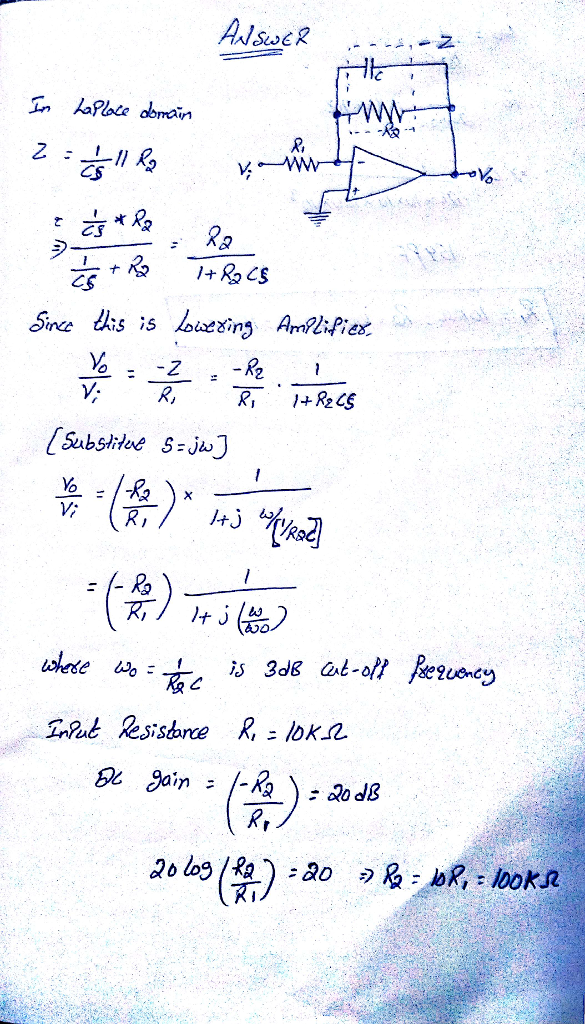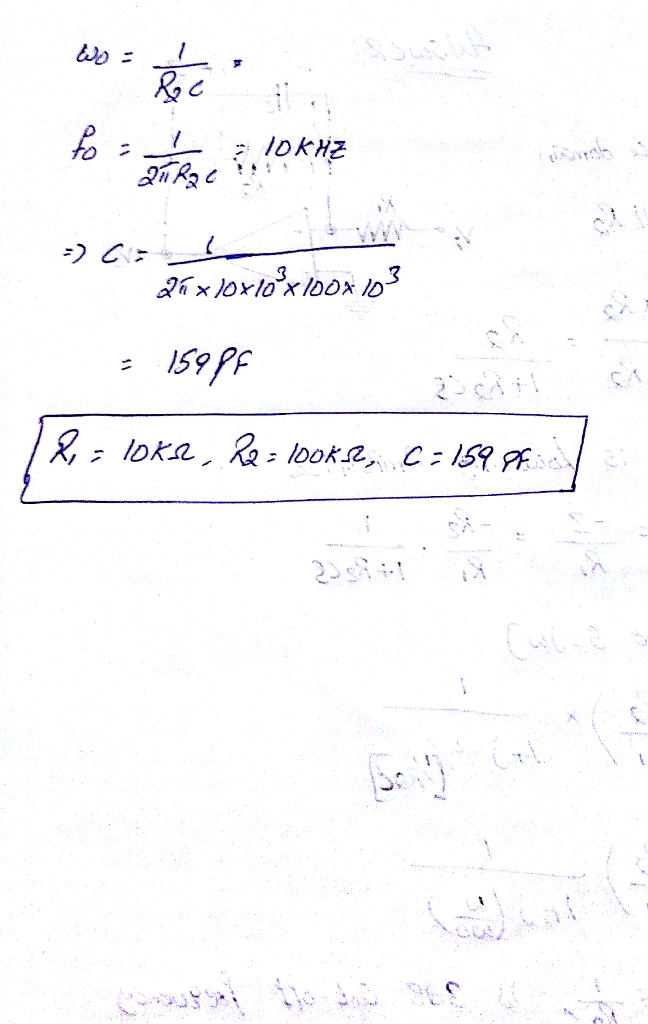# Homework Solution: Figure P2.86 shows a circuit that performs a low-pass STC function. Such a circuit is known as a first-order, low-pass active filte…

Figure P2.86 shows a circuit that performs a low-pass STC function. Such a circuit is known as a first-order, low-pass active filter. Derive the transfer function and show that the dc gain is (-R2/R1) and the 3-dB frequency wo=1/CR2. Design the circuit to obtain an input resistance of 10kOhms, a dc gain of 40 dB, and a 3-dB frequency of 1kHz. At what frequency does the magnitude of the transfer function reduce to unity? You should do this one using the "virtual ground" technique to keep the analysis simple. Please simulate the frequency response of the circuit in PSPICE and include an annotated graph and write some observations about it.